# Mechanical Engineering - Industrial Engineering and Production Management

### Exercise :: Industrial Engineering and Production Management - Section 1

26.

The determination of standard time in a complex job system is best done through

 A. stop watch time study B. analysis of micromotions C. grouping timing technique D. analysis of standard data system

Explanation:

No answer description available for this question. Let us discuss.

27.

In CPM, the cost slope is determined by

 A.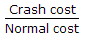B.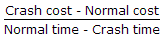C.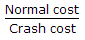D.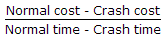Explanation:

No answer description available for this question. Let us discuss.

28.

If (R) is the base rate guaranteed per hour, (S) is the standard time for the job and (T) is the actual time, then according to Rowan plan, wages for the job will be

 A. TR B.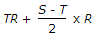C. TR + (S - T)R D.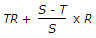Explanation:

No answer description available for this question. Let us discuss.

29.

In a line organisation

 A. responsibility of each individual is fixed B. discipline is strong C. quick decisions are taken D. all of these

Explanation:

No answer description available for this question. Let us discuss.

30.

Which of the following are the guidelines for the construction of a network diagram?

 A. Each activity is represented by one and only one arrow in the network. B. Dangling must be avoided in a network diagram. C. Dummy activity consumes no time or resource. D. all of the above